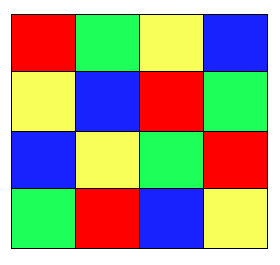#### You may also like### DOTS Division

Take any pair of two digit numbers x=ab and y=cd where, without loss of generality, ab > cd . Form two 4 digit numbers r=abcd and s=cdab and calculate: {r^2 - s^2} /{x^2 - y^2}.### Novemberish

a) A four digit number (in base 10) aabb is a perfect square. Discuss ways of systematically finding this number. (b) Prove that 11^{10}-1 is divisible by 100.### 2-digit Square

A 2-Digit number is squared. When this 2-digit number is reversed and squared, the difference between the squares is also a square. What is the 2-digit number?

# Latin Numbers

##### Age 14 to 16Challenge Level

Latin Numbers printable worksheet

Here is an example of a 4 by 4 Latin square:Can you see what is going on?

In a Latin Square each symbol or colour occurs exactly once in each row and exactly once in each column.

In the grid below, N is a 6 digit number with a very special property:
if you double the number and write it in the second row,
treble the number and write it in the third row,
and so on...
you end up with a Latin Square!

Can you find the six digit number N?

If you're finding it difficult to get started, click below to see a diagram showing one possible order in which you can work out each value.

Work out the number in the pale blue cell first.
Then the numbers in the column marked "2nd", then "3rd" and so on...

 1st 3rd 4th 5th 2nd

There is some more advice in the Getting Started section.